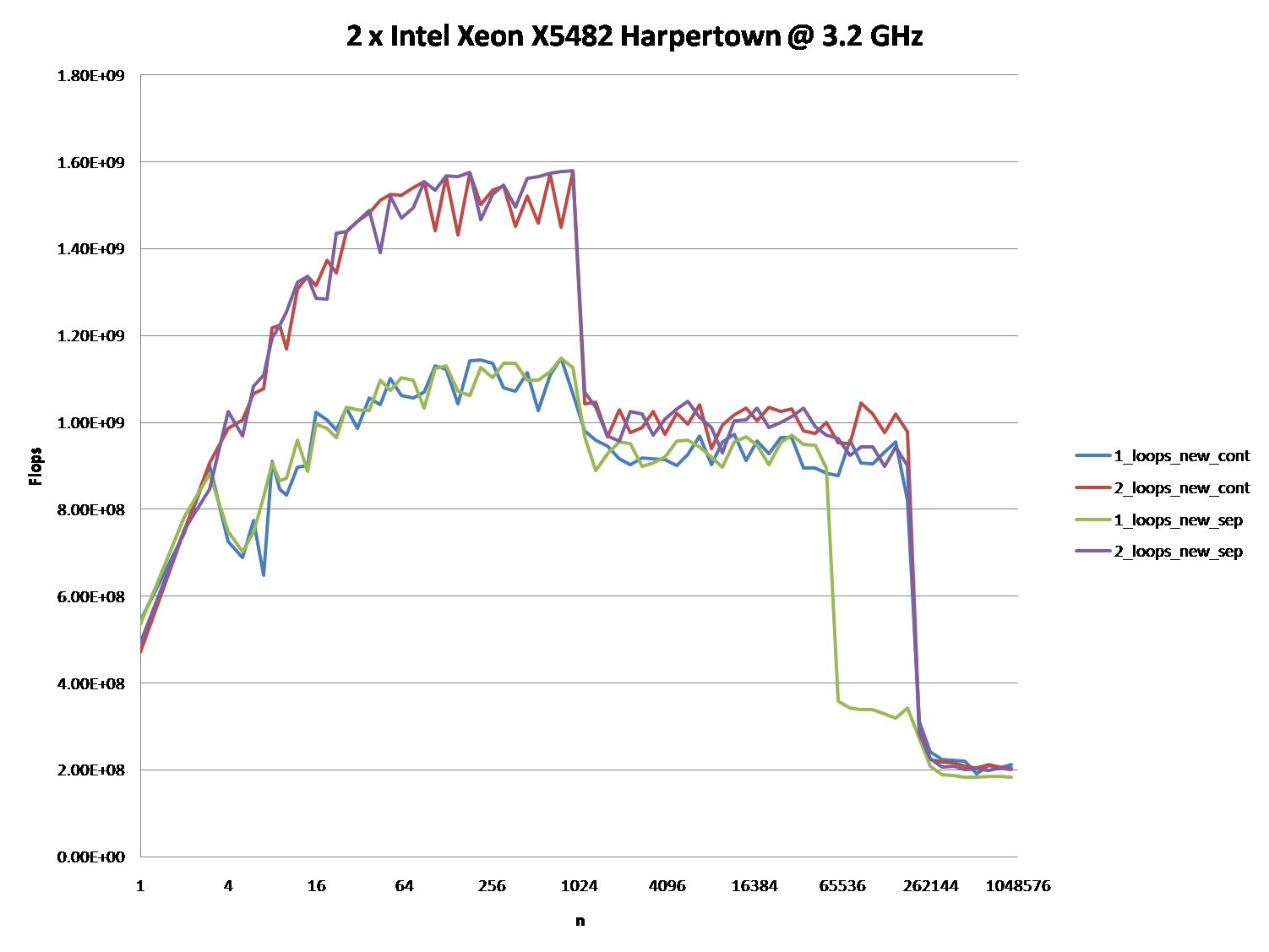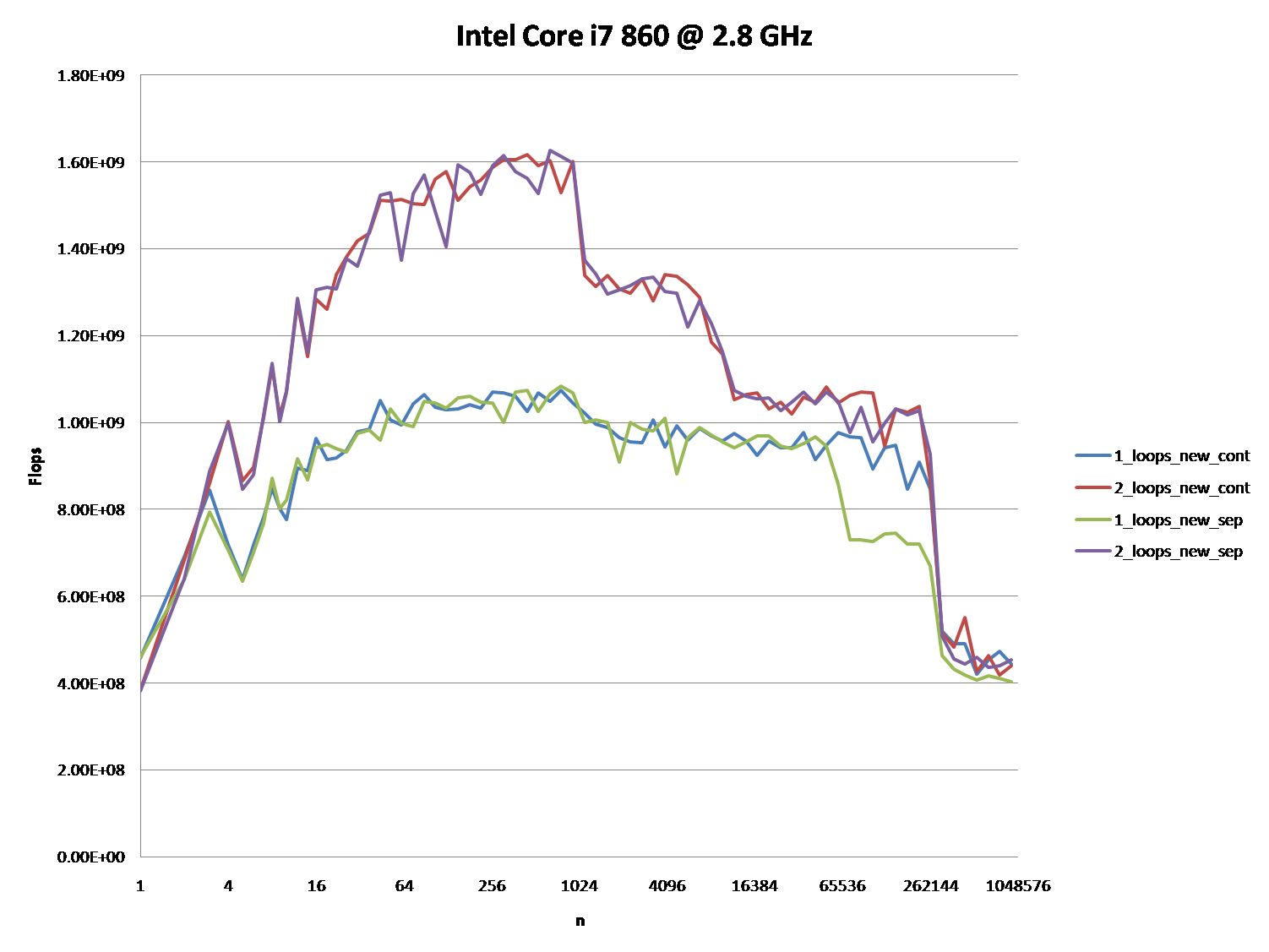# C++: Why is one loop so much slower than two loops?Suppose `a1`, `b1`, `c1`, and `d1` point to heap memory and my numerical code has the following core loop.

``````const int n=100000

for(int j=0;j<n;j++){
a1[j] += b1[j];
c1[j] += d1[j];
}``````

This loop is executed 10,000 times via another outer `for` loop. To speed it up, I changed the code to:

``````for(int j=0;j<n;j++){
a1[j] += b1[j];
}
for(int j=0;j<n;j++){
c1[j] += d1[j];
}``````

Compiled on MS Visual C++ 10.0 with full optimization and SSE2 enabled for 32-bit on a Intel Core 2Duo (x64), the first example takes 5.5 seconds and the double-loop example takes only 1.9 seconds. My question is: (Please refer to the my rephrased question at the bottom)

PS: I am not sure, if this helps:

Disassembly for the first loop basically looks like this (this block is repeated about five times in the full program):

``````movsd       xmm0,mmword ptr [edx+18h]
movsd       mmword ptr [ecx+20h],xmm0
movsd       xmm0,mmword ptr [esi+10h]
movsd       mmword ptr [eax+30h],xmm0
movsd       xmm0,mmword ptr [edx+20h]
movsd       mmword ptr [ecx+28h],xmm0
movsd       xmm0,mmword ptr [esi+18h]

Each loop of the double loop example produces this code (the following block is repeated about three times):

``````addsd       xmm0,mmword ptr [eax+28h]
movsd       mmword ptr [eax+28h],xmm0
movsd       xmm0,mmword ptr [ecx+20h]
movsd       mmword ptr [eax+30h],xmm0
movsd       xmm0,mmword ptr [ecx+28h]
movsd       mmword ptr [eax+38h],xmm0
movsd       xmm0,mmword ptr [ecx+30h]
movsd       mmword ptr [eax+40h],xmm0``````

EDIT: The question turned out to be of no relevance, as the behavior severely depends on the sizes of the arrays (n) and the CPU cache. So if there is further interest, I rephrase the question:

Could you provide some solid insight into the details that lead to the different cache behaviors as illustrated by the five regions on the following graph?

It might also be interesting to point out the differences between CPU/cache architectures, by providing a similar graph for these CPUs.

PPS: The full code is at http://pastebin.com/ivzkuTzG. It uses TBB Tick_Count for higher resolution timing, which can be disabled by not defining the TBB_TIMING Macro.

(It shows FLOP/s for different values of `n`.)Johannes Gerer

Upon further analysis of this, I believe this is (at least partially) caused by data alignment of the four pointers. This will cause some level of cache bank/way conflicts.

If I’ve guessed correctly on how you are allocating your arrays, they are likely to be aligned to the page line.

This means that all your accesses in each loop will fall on the same cache way. However, Intel processors have had 8-way L1 cache associativity for a while. But in reality, the performance isn’t completely uniform. Accessing 4-ways is still slower than say 2-ways.

EDIT : It does in fact look like you are allocating all the arrays separately. Usually when such large allocations are requested, the allocator will request fresh pages from the OS. Therefore, there is a high chance that large allocations will appear at the same offset from a page-boundary.

Here’s the test code:

``````int main(){
const int n = 100000;

#ifdef ALLOCATE_SEPERATE
double *a1 = (double*)malloc(n * sizeof(double));
double *b1 = (double*)malloc(n * sizeof(double));
double *c1 = (double*)malloc(n * sizeof(double));
double *d1 = (double*)malloc(n * sizeof(double));
#else
double *a1 = (double*)malloc(n * sizeof(double) * 4);
double *b1 = a1 + n;
double *c1 = b1 + n;
double *d1 = c1 + n;
#endif

//  Zero the data to prevent any chance of denormals.
memset(a1,0,n * sizeof(double));
memset(b1,0,n * sizeof(double));
memset(c1,0,n * sizeof(double));
memset(d1,0,n * sizeof(double));

cout << a1 << endl;
cout << b1 << endl;
cout << c1 << endl;
cout << d1 << endl;

clock_t start = clock();

int c = 0;
while (c++ < 10000){

#if ONE_LOOP
for(int j=0;j<n;j++){
a1[j] += b1[j];
c1[j] += d1[j];
}
#else
for(int j=0;j<n;j++){
a1[j] += b1[j];
}
for(int j=0;j<n;j++){
c1[j] += d1[j];
}
#endif

}

clock_t end = clock();
cout << "seconds = " << (double)(end - start) / CLOCKS_PER_SEC << endl;

system("pause");
return 0;
}``````

Benchmark Results:

# EDIT: Results on an actual Core 2 architecture machine:

2 x Intel Xeon X5482 Harpertown @ 3.2 GHz:

``````#define ALLOCATE_SEPERATE
#define ONE_LOOP
00600020
006D0020
007A0020
00870020
seconds = 6.206

#define ALLOCATE_SEPERATE
//#define ONE_LOOP
005E0020
006B0020
00780020
00850020
seconds = 2.116

//#define ALLOCATE_SEPERATE
#define ONE_LOOP
00570020
00633520
006F6A20
007B9F20
seconds = 1.894

//#define ALLOCATE_SEPERATE
//#define ONE_LOOP
008C0020
00983520
00A46A20
00B09F20
seconds = 1.993``````

Observations:

• 6.206 seconds with one loop and 2.116 seconds with two loops. This reproduces the OP’s results exactly.
• In the first two tests, the arrays are allocated separately. You’ll notice that they all have the same alignment relative to the page.
• In the second two tests, the arrays are packed together to break that alignment. Here you’ll notice both loops are faster. Furthermore, the second (double) loop is now the slower one as you would normally expect.

As @Stephen Cannon points out in the comments, there is very likely possibility that this alignment causes false aliasing in the load/store units or the cache. I Googled around for this and found that Intel actually has a hardware counter for partial address aliasing stalls:

# 5 Regions – Explanations

Region 1:

This one is easy. The dataset is so small that the performance is dominated by overhead like looping and branching.

Region 2:

Here, as the data sizes increases, the amount of relative overhead goes down and the performance “saturates”. Here two loops is slower because it has twice as much loop and branching overhead.

I’m not sure exactly what’s going on here… Alignment could still play an effect as Agner Fog mentionscache bank conflicts. (That link is about Sandy Bridge, but the idea should still be applicable to Core 2.)

Region 3:

At this point, the data no longer fits in L1 cache. So performance is capped by the L1 <-> L2 cache bandwidth.

Region 4:

The performance drop in the single-loop is what we are observing. And as mentioned, this is due to the alignment which (most likely) causes false aliasingstalls in the processor load/store units.

However, in order for false aliasing to occur, there must be a large enough stride between the datasets. This is why you don’t see this in region 3.

Region 5:

At this point, nothing fits in cache. So you’re bound by memory bandwidth.Mysticial

Stack Overflow